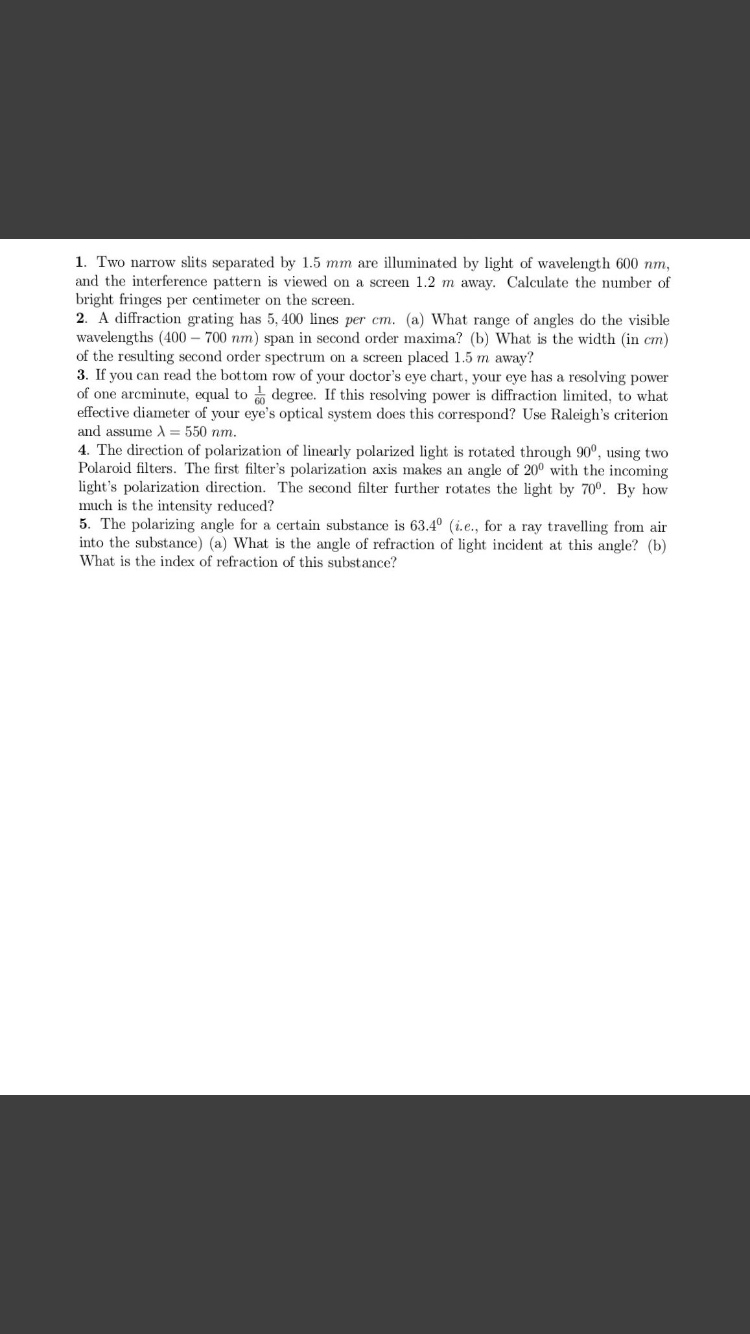# 1. Two narrow slits separated by 1.5 mm are illuminated by light of wavelength 600 nm,and the interference pattern is viewed on a screen 1.2 m away. Calculate the number ofbright fringes per centimeter on the screen.2. A diffraction grating has 5, 400 lines per cm. (a) What range of angles do the visiblewavelengths (400 – 700 nm) span in second order maxima? (b) What is the width (in cm)of the resulting second order spectrum on a screen placed 1.5 m away?3. If you can read the bottom row of your doctor's eye chart, your eye has a resolving powerof one arcminute, equal to degree. If this resolving power is diffraction limited, to whateffective diameter of your eye's optical system does this correspond? Use Raleigh's criterionand assume A = 550 nm.4. The direction of polarization of linearly polarized light is rotated through 90°, using twoPolaroid filters. The first filter's polarization axis makes an angle of 20° with the incominglight's polarization direction. The second filter further rotates the light by 70º. By howmuch is the intensity reduced?5. The polarizing angle for a certain substance is 63.4° (i.e., for a ray travelling from airinto the substance) (a) What is the angle of refraction of light incident at this angle? (b)What is the index of refraction of this substance?

Question
8 viewshelp_outlineImage Transcriptionclose1. Two narrow slits separated by 1.5 mm are illuminated by light of wavelength 600 nm, and the interference pattern is viewed on a screen 1.2 m away. Calculate the number of bright fringes per centimeter on the screen. 2. A diffraction grating has 5, 400 lines per cm. (a) What range of angles do the visible wavelengths (400 – 700 nm) span in second order maxima? (b) What is the width (in cm) of the resulting second order spectrum on a screen placed 1.5 m away? 3. If you can read the bottom row of your doctor's eye chart, your eye has a resolving power of one arcminute, equal to degree. If this resolving power is diffraction limited, to what effective diameter of your eye's optical system does this correspond? Use Raleigh's criterion and assume A = 550 nm. 4. The direction of polarization of linearly polarized light is rotated through 90°, using two Polaroid filters. The first filter's polarization axis makes an angle of 20° with the incoming light's polarization direction. The second filter further rotates the light by 70º. By how much is the intensity reduced? 5. The polarizing angle for a certain substance is 63.4° (i.e., for a ray travelling from air into the substance) (a) What is the angle of refraction of light incident at this angle? (b) What is the index of refraction of this substance? fullscreen
check_circle

Step 1

The Rayleigh criterion for vision that is diffraction...

### Want to see the full answer?

See Solution

#### Want to see this answer and more?

Solutions are written by subject experts who are available 24/7. Questions are typically answered within 1 hour.*

See Solution
*Response times may vary by subject and question.
Tagged in